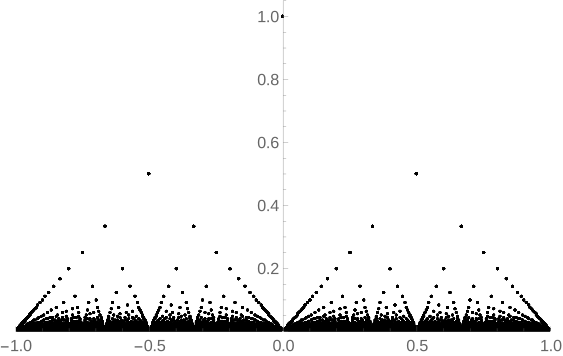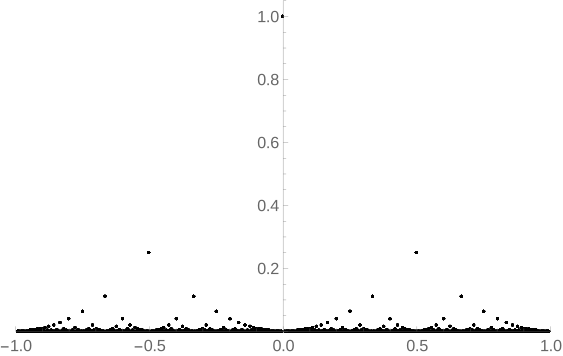# 浅谈扩展 Thomae 函数的可微性

$$f(x) = \begin{cases} 1/p & x \in \mathbb Q,\ x = q/p,\ p \in \mathbb N^+,\ q \in \mathbb Z,\ p \bot q \\ 0 & x \in \mathbb R \backslash \mathbb Q \end{cases}$$

$$f_k(x) = \begin{cases} 1/p^k & x \in \mathbb Q,\ x = q/p,\ p \in \mathbb N^+,\ q \in \mathbb Z,\ p \bot q \\ 0 & x \in \mathbb R \backslash \mathbb Q \end{cases}$$(Fig.1. $f_1(x)$$(-1,\ 1) 上的图像)(Fig.2. f_2(x)$$(-1,\ 1)$ 上的图像)

## 连续性

$$\{ap\} \geqslant 1 - \frac1p = \frac{p-1}p \tag{1}$$

$\alpha$ 为实数，对于任意正整数 $n$，总存在整数 $q$$[1,\ n] 内的正整数 p，满足：$$ |p\alpha - q| < \frac1n $$也就是：$$ \left|\alpha - \frac{q}p\right| < \frac1{np} \leqslant \frac1{p^2} $$虽然说还有更强的结论，但 Dirichlet 近似定理给人的感觉已经足够厉害并且形式简单。它的证明也相当初等：只需要用到鸽巢原理。直观上理解，|p\alpha - q| 这个东西的最小值就是 \min\{\{p\alpha\},\ 1 - \{p\alpha\}\}，因此目标就是证明存在 p \leqslant n 使得这个东西小于 1/n。如果存在 p 使得 \{p\alpha\} 小于 1/n 或者大于 1 - 1/n，那么结论直接成立。那有可能没有吗？考虑 \{0\cdot \alpha\}$$\{1\cdot \alpha\}$、…、$\{n\alpha\}$ 一共 $n + 1$ 个值，同时将区间 $[0,\ 1)$ $n$ 等分为 $[0,\ 1/n)$$[1/n,\ 2/n)、…、[(n-1)/n,\ 1)，根据鸽巢原理，必定存在 0 \leqslant i < j \leqslant n，使得 \{i\alpha\}$$\{j\alpha\}$ 在同一个区间里面。于是乎：

$$|\{j\alpha\} - \{i\alpha\}| < 1/n \tag{2}$$

$${f_2(x) \over x_n - a} \leqslant {1/p^2 \over q/p - a} \leqslant -1$$

$$ap - \frac1p \leqslant q < ap$$

## 参考资料

[DAP].Dirichlet’s Approximation Theorem
[BRS09]. Kevin Beanland, James W. Roberts, Craig Stevenson, “Modifications of Thomae’s Function and Differentiability” (2009), The Mathematical Association of America Monthly 116
[AoPS].Rational approximation”, Art of Problem Solving Wiki

1. 因为我们没有要求 $p$$q$ 互质，所以不能直接说等于。

2. $\{x\}$ 表示实数 $x$ 的小数部分，即 $x - \lfloor x \rfloor$。注意当 $x$ 是负数时的情况，如 $\{-0.3\} = -0.3 - (-1) = 0.7$ 而不是 $0.3$。

3. 对于有理数就不一样了，这种近似是有限的。这个结论在参考资料 [DAP] 的 “定理 2” 中证明了。

4. 参见 Equidistribution Theorem (Wikipedia)

5. 头一回听上去挺奇怪的，毕竟每个闭区间里面都有无数的有理数 -.-

6. 实际上这里的讨论只用到了 $f_k(x)$ 在有理点取正数这个性质，因此所有满足有理点取正数，无理点为 $0$ 的函数 $f(x)$ 都有这个性质。这也是 [BRS09] 的“命题 3.1”。